Courses

# Test: Level - 1 Electrochemistry - 1

## 25 Questions MCQ Test Chemistry Class 12 | Test: Level - 1 Electrochemistry - 1

Description
This mock test of Test: Level - 1 Electrochemistry - 1 for Class 12 helps you for every Class 12 entrance exam. This contains 25 Multiple Choice Questions for Class 12 Test: Level - 1 Electrochemistry - 1 (mcq) to study with solutions a complete question bank. The solved questions answers in this Test: Level - 1 Electrochemistry - 1 quiz give you a good mix of easy questions and tough questions. Class 12 students definitely take this Test: Level - 1 Electrochemistry - 1 exercise for a better result in the exam. You can find other Test: Level - 1 Electrochemistry - 1 extra questions, long questions & short questions for Class 12 on EduRev as well by searching above.
QUESTION: 1

### According to Faraday’s Second Law of Electrolysis the amounts of different substances liberated by the same quantity of electricity passing through the electrolytic solution are proportional

Solution:

The amounts of different substances liberated by the same quantity of electricity passing through the electrolytic solution are proportional to their chemical equivalent weights.

QUESTION: 2

### Charge on one mole of electrons - Faraday is approximately

Solution:

Charge on one electron is 1.6 x 10-19 C. 1 mole of electron 6.023 x 1023 electrons. So charge on one electron is = 1.6 x 10-19 C x 6.023 x 1023 = 96500 C mol-1

QUESTION: 3

### In the primary batteries

Solution:

Primary batteries cannot be recharged and reused.

QUESTION: 4

A secondary cell after use ________ recharged by passing current through it in the ________ so that it can/can’t be used again.

Solution:

Secondary cell can be recharged and reused.

QUESTION: 5

Fuel cells are ________ that are designed to convert the energy of combustion of fuels like hydrogen, methane, methanol, etc. directly into_________.

Solution:

Fuel Cells converts energy coming from the combustion of fuels directly to electrical energy.

QUESTION: 6

In corrosion a metal is ______ of electrons to oxygen and formation of oxides

Solution:

Metals is oxidized by loss of electrons on corrosion.

QUESTION: 7

One of the simplest methods of preventing corrosion is to prevent the surface of the metallic object to come in contact with atmosphere. This can be done

Solution:

Best way to prevent corrosion is to prevent the surface of metallic object to come in contct with atmosphere.

QUESTION: 8

An electrochemical method is to provide a sacrificial electrode of another metal (like Mg, Zn, etc.) which corrodes itself but saves the object. A typical example is

Solution:

Galvanization is a process in which iron is coated with reactive metal like Zn which get corroded hence preventing iron from corrosion.

QUESTION: 9

Correct arrangement of Al, Cu, Fe, Mg and Zn in the order in which they displace each other from the solution of their salts is

Solution:

Reactivity series.

QUESTION: 10

The standard emf of galvanic cell involving 3 moles of electrons in its redox reaction is 0.59 V. The equilibrium constant for the reaction of the cell is

Solution:
QUESTION: 11

Emf of the cell

Mg (s) | Mg2+ (0.001M) || Cu2+ (0.0001M) | Cu(s)   at 298 K is

Solution: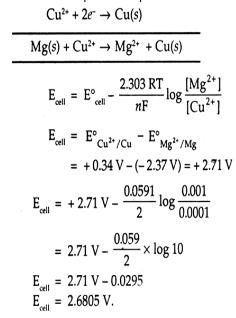QUESTION: 12

Emf of the cell

Fe (s) | Fe2+ (0.001 M)|| H+ (1M)|H2 (g) (1 bar) |Pt(s)   at 298 K is

Solution: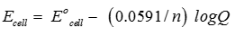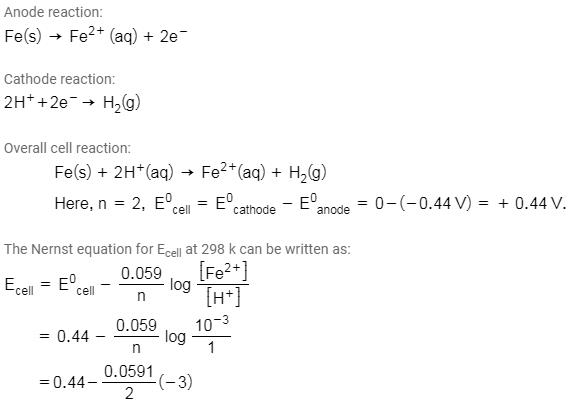QUESTION: 13

The conductivity of 0.20 M solution of KCl at 298 K is 0.0248 S  cm-1. Calculate its molar conductivity.

Solution: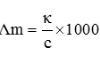QUESTION: 14

The resistance of a conductivity cell containing 0.001M KCl solution at 298 K is 1500 Ω. What is the cell constant if conductivity of 0.001M KCl solution at 298 K is 0.146 × 10-3 S cm-1.

Solution:

Κ = G x cell constant and G = 1/R.

QUESTION: 15

How much charge is required for the reduction of 1 mol of Al3+ to Al?

Solution:

For reduction of 1 mol of Al3+ to Al, 3 mol of electrons are required so total charge will be 3F.

QUESTION: 16

How much electricity in terms of Faraday is required to produce 40.0 g of Al from molten  Al2O3?

Solution:

Moles of Al = 40/27. For 1 mol of Al deposition 3F charge is required.

QUESTION: 17

Three electrolytic cells A, B, C containing solutions of ZnSO4, AgNO3 and CuSO4, respectively are connected in series. A steady current of 1.5 amperes was passed through them until 1.45 g of silver deposited at the cathode of cell. How long did the current flow? What mass of copper and zinc were deposited?

Solution: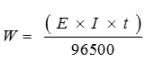And mass of Zn and Cu deposited will be in ratio of their equivalent mass.

QUESTION: 18

The standard emf of galvanic cell involving 3 moles of electrons in its redox reaction is 0.59 V. The equilibrium constant for the reaction of the cell is

Solution: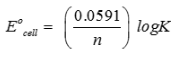QUESTION: 19

An increase in equivalent conductance of a strong electrolyte with dilution is mainly due to

Solution:

Equivalent conductance increases on dilution for a strong electrolyte because of increase in mobility of ions.

QUESTION: 20

For the reduction of silver ions with copper metal the standard cell potential was found to be +0.46V at 25°C. The value of standard Gibbs energy, ΔG° wll be (F = 96500 C mol-1)

Solution: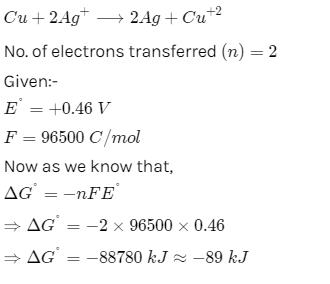QUESTION: 21

Which of the following electrolytic solutions has the least specific conductance?

Solution:

Specific conductance decreases with dilution because of decrease in the number of ions per unit volume.

QUESTION: 22

The highest electrical conductivity of the following aqueous solutions is of

Solution:

Acidity increases on attaching electron withdrawing group because of stability of conjugate base.

QUESTION: 23

The standard electrode potential is measured by

Solution:

Voltmeter measures the potential.

QUESTION: 24

When KMnO4 acts as an oxidizing agent and ultimately forms MnO42-, MnO2, Mn2O3, and Mn2+ then the number of electrons transferred in each case

Solution:

No. of electrons = change in oxidation number of active elemnt.

QUESTION: 25

On the basis of the following E° values, the strongest oxidizing agent is: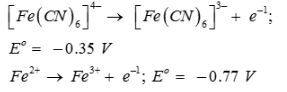Solution:

Strongest oxidizing agent is one having more positive or less negative reduction potential.